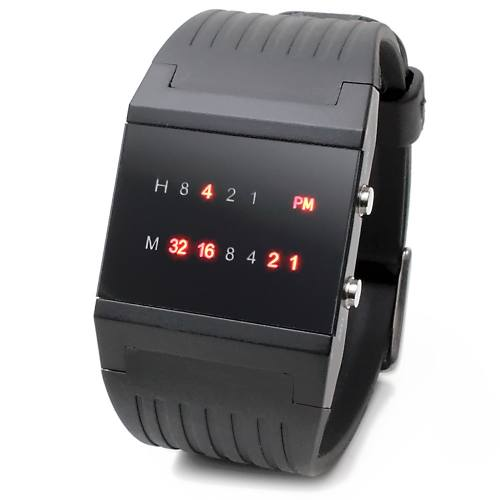# Leetcode 401 - Binary watch

Note:

• Bitwise

• It’s a binary watch. Hour is represented by 4 bits. Min is represented by 6 bits.
• The number of 1s in each binary number means how many LEDs are on.
• Too many cases, using backtracking is good for finding out all combinations.

• Need to seperately call dfs on hour[] and min[].

• Use two tmp arrays to contain H/M, then use a double for loop to get all combinations.

Question:

A binary watch has 4 LEDs on the top which represent the hours (0-11), and the 6 LEDs on the bottom represent the minutes (0-59). Each LED represents a zero or one, with the least significant bit on the right.

• For example, the below binary watch reads “4:51”.Given an integer turnedOn which represents the number of LEDs that are currently on, return all possible times the watch could represent. You may return the answer in any order.

The hour must not contain a leading zero.

• For example, “01:00” is not valid. It should be “1:00”.
The minute must be consist of two digits and may contain a leading zero.

• For example, “10:2” is not valid. It should be “10:02”.

Example:

Code:

BitWise

DFS + Backtracking